##### Pre-Calculus: 1001 Practice Problems For Dummies (+ Free Online Practice)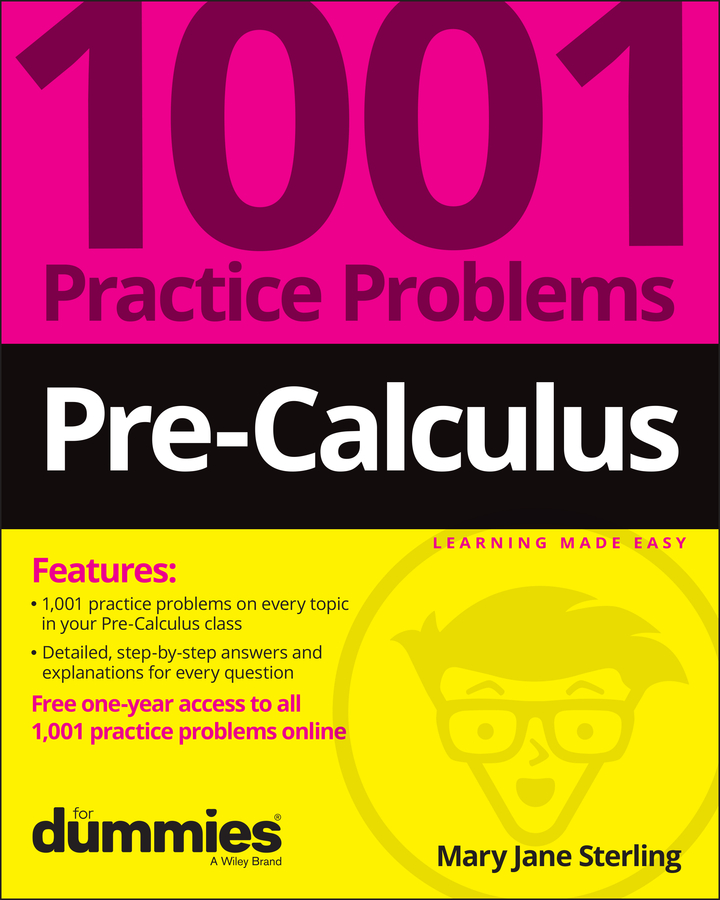When solving linear systems, you have two methods — substitution or elimination — at your disposal, and which one you choose depends on the problem. If the coefficient of any variable is 1, which means you can easily solve for it in terms of the other variable, then substitution is a very good bet. If all the coefficients are anything other than 1, then you can use elimination, but only if the equations can be added together to make one of the variables disappear.

## How to solve linear systems with the substitution method

In the substitution method, you use one equation to solve for one variable and then substitute that expression into the other equation to solve for the other variable. To begin the easiest way, look for a variable with a coefficient of 1 and solve for it. You just have to add or subtract terms in order to move everything to the other side of the equal sign, just like you would normally do to solve for variables. That way, you won't have to divide by the coefficient when you're solving, which means you won't have any fractions (unless there are already fractions to begin with).

For example, suppose you're managing a theater and you need to know how many adults and children are in attendance at a show. The auditorium is sold out and contains a mixture of adults and children. The tickets cost \$23.00 per adult and \$15.00 per child. If the auditorium has 250 seats and the total ticket revenue for the event is \$4,846.00, how many adults and children are in attendance?

To solve the problem with the substitution method, follow these steps:

1. Express the word problem as a system of equations.

You want to solve for how many adult tickets (a) and child tickets (c) you sold. If the auditorium has 250 seats and was sold out, the sum of the adult tickets and child tickets must be 250.

The ticket prices also lead you to the revenue (or money made) from the event. The adult ticket price times the number of adults present lets you know how much money you made from the adults. You can do the same calculation with the child tickets. The sum of these two calculations must be the total ticket revenue for the event.

Here's how you write this system of equations: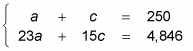2. Solve for one of the variables.

Pick the variable with a coefficient of 1 if you can, because solving for this variable will be easy. For this example, you can choose to solve for a in the first equation. To do so, subtract c from both sides: a = 250 – c.

3. Substitute the solved variable into the other equation.

In this example, you solve for a in the first equation. You take this value (250 – c) and substitute it into the other equation for a. (Make sure that you don't substitute into the equation you used in Step 1; otherwise, you'll be going in circles.)

The second equation now says 23(250 – c) + 15c = 4,846.

4. Solve for the unknown variable.

You distribute the number 23:

5,750 – 23c + 15c = 4,846

And then you simplify:

5,750 – 8c = 4,846, or –8c = –904

So c = 113. A total of 113 children attended the event.

5. Substitute the value of the unknown variable into one of the original equations to solve for the other unknown variable.

When you plug 113 into the first equation for c, you get a + 113 = 250. Solving this equation, you get a = 137. You sold a total of 137 adult tickets.

6. Check your solution.

When you plug a and c into the original equations, you should get two true statements. Does 137 + 113 = 250? Yes. Does 23(137) + 15(113) = 4,846? Indeed.

## How to solve linear systems with the elimination method

If solving a system of two equations with the substitution method proves difficult or the system involves fractions, the elimination method is your next best option. In the elimination method, you make one of the variables cancel itself out by adding the two equations.

Sometimes you have to multiply one or both equations by constants in order to add the equations; this situation occurs when you can't eliminate one of the variables by just adding the two equations together. (Remember that in order for one variable to be eliminated, the coefficients of one variable must be opposites.)

For example, the following steps show you how to solve this system by using the process of elimination: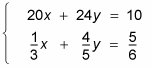1. Rewrite the equations, if necessary, to make like variables line up underneath each other.

The order of the variables doesn't matter; just make sure that like terms line up with like terms from top to bottom. The equations in this system have the variables x and y lined up already: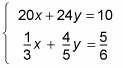2. Multiply the equations by constants to make one set of variables match coefficients.

First, leave the top equation alone and multiply the bottom equation by 30 (to eliminate all denominators). (Be sure to distribute this number to each term — even on the other side of the equal sign.) Doing this step gives you the following equations: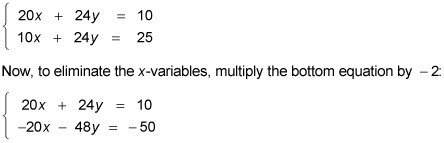3. Add the two equations.

You now have –24y = –40.

4. Solve for the unknown variable that remains.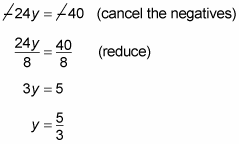5. Substitute the value of the found variable into either equation.

This example uses the first equation: 20x + 24(5/3) = 10.

6. Solve for the final unknown variable.

You end up with x = –3/2.

7. Check your solutions.

Always verify your answer by plugging the solutions back into the original system. These check out!

20(–3/2) + 24(5/3) = –30 + 40 = 10

It works! Now check the other equation: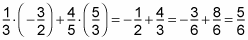Because both values are solutions to both equations, the solution to the system is correct.